Learn data science and machine learning by building real-world projects on Jovian

## Project Whatsapp Message Exploratory Data Analysis(EDA)

Author: Michael Chia Yin

## Out-line

• Introduction.
• Data Retrieval.
• Data Preparation and Cleaning.
• Exploratory Data Analysis(EDA)
• Conclusion.

## Introduction:

Hello there, thanks for review my notebook! So today we are going to analyse the whatsapp chat that we normally use everydays. In here we are going to use a chat group that is "University group" and in this group we normally will exchage knowleged that we learn by teaching one and others.

Just some informations this group was created early this year that was around 05/02/2020 til 21/09/2020 period. So let us dive in one what we are going to discover!

You can read up my medium post : https://medium.com/@edsenmichaelcy/whatsapp-message-exploratory-data-analysis-eda-538560ee1c99

## Data Retrieval

First before any EDA to be done we must first understand how to get the data we need. Normally we can go to kaggle.com to start getting our dataset. But for this EDA we will use the whatsapp data that everyone can export in they own whatsapp group. Let me show how you are able to retrieval the data easily.

Now what you need to do is just click on More, and Export Chat .### Take note

I am export without media because if the media files is more than a certain value then not all the files are able to be exportedAfter exporting the file, you now able to view the text file format### Install all the important libraries for this project

In :
``````!pip install jovian --upgrade --quiet
``````
In :
``````project_name = "whatsapp-chat-analysis-course-project"

``````
In :
``import jovian``
In [ ]:
``jovian.commit(project=project_name,enviroment=None,files=["Chat.txt"])``
```[jovian] Attempting to save notebook.. ```

## Data Preparation and Cleaning

Before we start our data preparation and cleaning there are so few item we need to take noted:

1. Business and data understand is the success key factor of a good analysis
2. Make sure all the row number is balance because we did now want to see outliner
3. Make sure the data are clean, such as you all need text not picture.

### Import libraries

In this project we will using some unique libraries such as below:

Regex(re):

• This libraries is use to extract and manipulate string based on specific patterns

Pandas

• We will use pandas to process the data and do basic analysis

Matlotlib,seaborn & plotly

• We are going to use this libraries as ours tools for data visualization

Emojis

• The Emojis libraries normally handle emojis in the text. It was a great libraries for pytho.

wordcloud

• This invlove creating word that are related pattern for the most use word.
In :
``````import plotly.express as px
import os
import pandas as pd
import re
import datetime as time
import jovian
import numpy as np
import matplotlib.pyplot as plt
import matplotlib
import seaborn as sns
import emoji
import re
from collections import Counter
from wordcloud import WordCloud, STOPWORDS``````

Before we start any analysis we need to understand the Business and the data side

1. In any project we must understand what exactly we want to found out in this project?
2. Do this analysis help us to achieve business goal?

Data Understanding

1. In here we can see there are 3 columns in the dataset
2. The dataset contains date, text and a NaN value
3. Using the info(), we are able to know the row of each object is not balance because there are 21k message but some of the columns only have 23k and 700
4. After knowing there is an unknown value in the dataset and inbalance row we now can clear the data
In :
``````whatsapp_df = pd.read_fwf('Chat.txt', header = None)

whatsapp_df
``````
Out:
In :
``whatsapp_df.info()``
```<class 'pandas.core.frame.DataFrame'> RangeIndex: 23330 entries, 0 to 23329 Data columns (total 3 columns): # Column Non-Null Count Dtype --- ------ -------------- ----- 0 0 23177 non-null object 1 1 23087 non-null object 2 2 788 non-null object dtypes: object(3) memory usage: 546.9+ KB ```

After that we will use the info() that provided by the pandas to understand the datatype in the dataframe. As you can see we need to do some clearning such as the date and the Media omitted. (re-explain)

In :
``whatsapp_df.shape``
Out:
``(23330, 3)``

So now we understand the column name need to be changed instead of using 0,1 and 2 we need to change it to more meaningful name such as DateTime, user and messages then we will put it as whatsapp_df. Also, we want to make all the row and column are in the same value. In this project, you will notice that I will be repeating using whatsapp_df and copy into multiple data frame.

In :
``````def txtTodf(txt_file):
'''Convert WhatsApp chat log text file to a Pandas dataframe.'''

# some regex to account for messages taking up multiple lines
pat = re.compile(r'^(\d\d\/\d\d\/\d\d\d\d.*?)(?=^^\d\d\/\d\d\/\d\d\d\d|\Z)', re.S | re.M)
with open(txt_file) as file:
data = [m.group(1).strip().replace('\n', ' ') for m in pat.finditer(file.read())]

user     = [];
message  = [];
datetime = []

for row in data:

# timestamp is before the first dash
datetime.append(row.split(' - '))

# sender is between am/pm, dash and colon
try:
s = re.search('m - (.*?):', row).group(1)
user.append(s)
except:
user.append('')

# message content is after the first colon
try:
message.append(row.split(': ', 1))
except:
message.append('')

df = pd.DataFrame(zip(datetime, user, message), columns=['datetime', 'user', 'message'])
df['datetime'] = pd.to_datetime(df.datetime, format='%d/%m/%Y, %I:%M %p')

# remove events not associated with a sender
df = df[df.user != ''].reset_index(drop=True)

return df

whatsapp_df = txtTodf('Chat.txt')``````

After clearning the data, now you are able to see the dataframe/tables is more easy to read than the pervious tables.

In :
``whatsapp_df.head(10)``
Out:

Now in the info you are able to see all the row is now balance as it show all is 22k

In :
``whatsapp_df.info()``
```<class 'pandas.core.frame.DataFrame'> RangeIndex: 22701 entries, 0 to 22700 Data columns (total 3 columns): # Column Non-Null Count Dtype --- ------ -------------- ----- 0 datetime 22701 non-null datetime64[ns] 1 user 22701 non-null object 2 message 22701 non-null object dtypes: datetime64[ns](1), object(2) memory usage: 532.2+ KB ```

We will now save our work by using jovian.commit

In [ ]:
``jovian.commit(project=project_name,enviroment=None,files=["Chat.txt"])``
```[jovian] Attempting to save notebook.. ```

## Cleaning the image data

After we are done cleaning the columns data, now we must make sure to clear all the image/media data because we are not going use that as our data analysis questions. Since we want to do analysis on the text rather than the image so we have to clean the image data in the text file. In here we have 11k of the image in the three-row. The below diagram is showing how to drop the image file.

In :
``````# To understand the number od the image data
img = whatsapp_df[whatsapp_df['message'] == "<Media omitted>" ]
img.shape

``````
Out:
``(1182, 3)``

So now we will drop all the img to make the dataset more clean. Moreover, we want to make sure it will not copy a new dataset that why we will use "inplace == True"

In :
``````# We will drop all the image file by using the Drop functions
whatsapp_df.drop(img.index, inplace=True)``````

As you can see now the dataset is clean from the media format.But we have a problem because after we did the clearning the index of the dataset had been off-order. So now we have to clean the data by using the reset_index().

In :
``whatsapp_df.head(10)``
Out:

So after the data is clean we have left 21519 data in our dataset. So now we are able to perform the data driven decision making!

In :
``````whatsapp_df.reset_index(inplace=True, drop=True)
whatsapp_df.shape
``````
Out:
``(21519, 3)``

## Let get started on the Exploratory Data Analysis(EDA)

1. Which users have the most Chat/messages in the group?
2. Which emojis use the most by which users?
3. Most active hours?
4. Which month have the highest messages and also the busiest month?
5. Determine which word or text did the user use the most?

To get more details explanation you can visit my medium link:??

In [ ]:
``jovian.commit(project=project_name,enviroment=None,files=["Chat.txt"])``
```[jovian] Attempting to save notebook.. ```

## 1. Which users have the most Chat/messages in the group?

In any WhatsApp analysis, we always want to know which user normally chat the most in the group. This help as we determine the most active person in the chat group.

In :
``````#Understand how many user and messages in this chat first
totalNumberofMessage = whatsapp_df.message.count()
username   = whatsapp_df["user"].unique() #unique is a functions that able find the unique elements of an array

print('The total of the number of message:',totalNumberofMessage)
print('User name that involve in the chat:',username)``````
```The total of the number of message: 21519 User name that involve in the chat: ['Ed' 'Rohit' 'Pei Yin'] ```

Now we will start creating a new dataframe by copying from the old dataframe, the reason is very simple because we did not want to edit the original dataframe.

In :
``````#Creating a new dataframe by copying the old dataframe
whatsapp_df1 = whatsapp_df.copy()
whatsapp_df1['Number_of_messages'] = * whatsapp_df1.shape
whatsapp_df1.drop(columns = 'datetime', inplace = True)

#We are groupby the user and messages together then we will use count() to count the messages for each of user
whatsapp_df1 = whatsapp_df1.groupby('user')['Number_of_messages'].count().sort_values(ascending = False).reset_index()
whatsapp_df1
``````
Out:

### We will use different data visualization method for this case

1. Plot Chart

2. Bar Chart

We are going to create a plot chart for our first data visualization method.

As you can see the results have shown us the most number of messages is by users call "Rohit" that is around 10k and this show "Rohit" is a very active member in the group

In :
``````#  Using seaborn for Styles
sns.set_style("darkgrid")

# Resize the  figure size
plt.figure(figsize=(12, 9))

# Here we are ploting the line chart using plt.plot
plt.plot(whatsapp_df1.user, whatsapp_df1.Number_of_messages, 'o--c')

# In here we are writing the Labels and Title for the plot chart
plt.xlabel('Users')
plt.ylabel('Total number of messages')

plt.title("The highest number of messages send by the user")
plt.legend(['Messages send']);

``````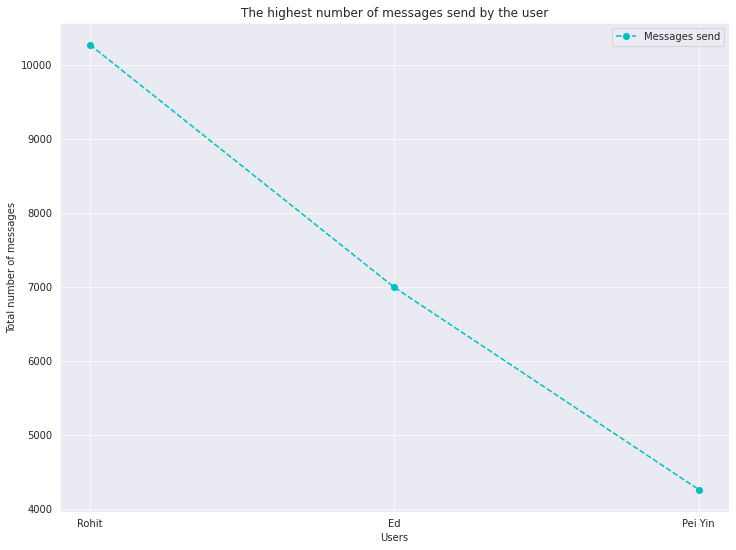In the previous plot we are using matplotlib. Now let use seaborn(sns) to beautify our chart and this time we will use bar chart as our data visualization

In :
``````#Formating
sns.set_style("darkgrid")

#The background of the chart
matplotlib.rcParams['font.size'] = 12
matplotlib.rcParams['figure.figsize'] = (12, 9)
matplotlib.rcParams['figure.facecolor'] = '#00000000'
fig, ax = plt.subplots()

#Creating a bar chart
sns.barplot(whatsapp_df1.user,whatsapp_df1.Number_of_messages,hue='user',data=whatsapp_df1,dodge=False,palette="CMRmap")

#The title of our charts
plt.title("The highest number of messages")

#Change the width of the bar chart plot
def change_width(ax, new_value) :
for patch in ax.patches :
current_width = patch.get_width()
diff = current_width - new_value

# we change the bar width
patch.set_width(new_value)

# we recenter the bar
patch.set_x(patch.get_x() + diff * .5)

change_width(ax, .35)
plt.show()

``````
```/srv/conda/envs/notebook/lib/python3.7/site-packages/seaborn/_decorators.py:43: FutureWarning: Pass the following variables as keyword args: x, y. From version 0.12, the only valid positional argument will be `data`, and passing other arguments without an explicit keyword will result in an error or misinterpretation. FutureWarning ```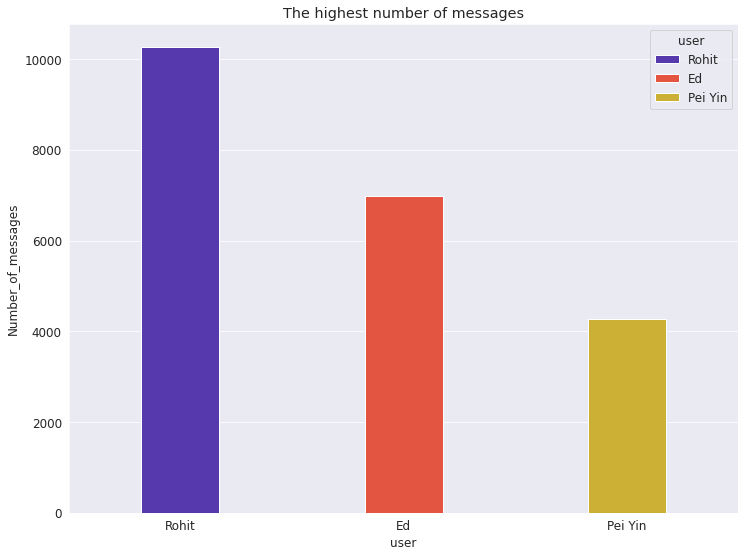## 2.Which emojis use the most by which users?

Now we want to know which emoji is used widely by the user and from the analysis, we can do an assumption that user will most likely to use emoji again in the other chat.

First we need to count the number of emoji in the mmessage row by using the UNICODE_EMOJI to search the code for the emoji

In :
``````#Copy a dataset
whatsapp_df2 = whatsapp_df.copy()

#Count the number of emoji
emoji_ctr = Counter()
emojis_list = map(lambda x: ''.join(x.split()), emoji.UNICODE_EMOJI.keys()) #UNICODE_EMOJI class have a thee emoji code inside
r = re.compile('|'.join(re.escape(p) for p in emojis_list))
for idx, row in whatsapp_df2.iterrows():
emojis_found = r.findall(row["message"]) #The findall() is a functions for regex that help to find a matches
for emoji_found in emojis_found:
emoji_ctr[emoji_found] += 1

``````

As you can see when already rendered the emoji from the whatsapp_df2 and also successfully put in the dataframe table.Now all we need to do is just put in the Pie chart as our data visualization.

In :
``````#This will help to create or rendered the emoji
emojis_df = pd.DataFrame() #The reason to use pd.dataframe is we want to put the emojis_df into the dataframe tables
emojis_df['emoji'] = [''] * 10
emojis_df['number_of_Emoji'] =  * 10

i = 0
for item in emoji_ctr.most_common(10):
emojis_df.emoji[i] = item
emojis_df.number_of_Emoji[i] = int(item)

i += 1

emojis_df
``````
```/srv/conda/envs/notebook/lib/python3.7/site-packages/ipykernel_launcher.py:8: SettingWithCopyWarning: A value is trying to be set on a copy of a slice from a DataFrame See the caveats in the documentation: https://pandas.pydata.org/pandas-docs/stable/user_guide/indexing.html#returning-a-view-versus-a-copy /srv/conda/envs/notebook/lib/python3.7/site-packages/ipykernel_launcher.py:9: SettingWithCopyWarning: A value is trying to be set on a copy of a slice from a DataFrame See the caveats in the documentation: https://pandas.pydata.org/pandas-docs/stable/user_guide/indexing.html#returning-a-view-versus-a-copy if __name__ == '__main__': ```
Out:

Before we go into each of the users to determine which emoji is widely used by the user. We need to look at the overall emoji that have been used from three of the users. As you can see on the results, the most widely use emoji among the three users is Face with Tears of Joy that stand around 79.7% from the overall. So we can agree that most of the time the user will use Face with Tears of Joy Emoji in this group chat

Bouns tips

We will use plotly to create our pie-charts for Emojis Learn more: https://plotly.com/python/pie-charts/

In :
``````#This pei chart give us and ideas the overall view of which emoji use the most
fig = px.pie(emojis_df, values='number_of_Emoji', names='emoji',title='Emoji percentage used in chat group')
fig.update_traces(textposition='inside', textinfo='percent+label')
fig.show()
``````

After knowing the Tears of Joy Emoji is the most widely use in the group chat. Now we want to understand each of the user use what emoji the most.

In :
``````#Now we want to know which emoji is use the most by each of the users. But since the first results only create
#emoji and number_emoji in the dataframe now we need to create a dataframe contain user and emojio they use

emoji_ctr = Counter()
emojis_list = map(lambda x: ''.join(x.split()), emoji.UNICODE_EMOJI.keys())
r = re.compile('|'.join(re.escape(p) for p in emojis_list))
for idx, row in whatsapp_df2.iterrows():
emojis_found = r.findall(row["message"])
for emoji_found in emojis_found:
emoji_ctr[emoji_found] += 1``````

This time we will not create a emoji_df because we want to use the user row and emoji row. So we are able to know each of the users use what emoji

In :
``````emojis_df = whatsapp_df2

emojis_df['emoji'] = [''] * 21519
emojis_df['number_of_Emoji'] =  * 21519

i = 0
for item in emoji_ctr.most_common(21519):
emojis_df.emoji[i] = item
emojis_df.number_of_Emoji[i] = int(item)

i += 1

emojis_df
``````
```/srv/conda/envs/notebook/lib/python3.7/site-packages/ipykernel_launcher.py:8: SettingWithCopyWarning: A value is trying to be set on a copy of a slice from a DataFrame See the caveats in the documentation: https://pandas.pydata.org/pandas-docs/stable/user_guide/indexing.html#returning-a-view-versus-a-copy /srv/conda/envs/notebook/lib/python3.7/site-packages/ipykernel_launcher.py:9: SettingWithCopyWarning: A value is trying to be set on a copy of a slice from a DataFrame See the caveats in the documentation: https://pandas.pydata.org/pandas-docs/stable/user_guide/indexing.html#returning-a-view-versus-a-copy ```
Out:

### In here we are able to see each of the users use what emoji the most

User: Ed

1. This user uses Woman Gesturing OK Emoji the most
2. The second is Tears of Joy Emoji

User : Rohit

1. This user has a diversities emoji use in the group chat, so we cannot determine what emoji he uses the most or use in the future.

User : Pei Yin

1. This user will use two emoji that are in common that is Thumbs Up and Clapping emoji
In :
``````l = emojis_df.user.unique()
for i in range(len(l)):
dummy_df = emojis_df[emojis_df['user'] == l[i]]
emojis_list = list([a for b in dummy_df.emoji for a in b])
emoji_dict = dict(Counter(emojis_list))
emoji_dict = sorted(emoji_dict.items(), key=lambda x: x, reverse=True)
print('Emoji Distribution for', l[i])
user_emoji_df = pd.DataFrame(emoji_dict, columns=['emoji', 'count'])
fig = px.pie(user_emoji_df, values='count', names='emoji')
fig.update_traces(textposition='inside', textinfo='percent+label')
fig.show()
``````
```Emoji Distribution for Ed ```
```Emoji Distribution for Rohit ```
```Emoji Distribution for Pei Yin ```

## 3. Most active hour in whatsapps

In this analysis, it helps us to understand what is the hours where all the member is very active in WhatsApp. We will depend on two variable on is the number of messages and the hours. Then we will able to know when is the most active hours

In :
``````#Copy a dataframe
whatsapp_df3 = whatsapp_df.copy()

whatsapp_df3['number_of_message'] =  * whatsapp_df3.shape

whatsapp_df3['hours'] = whatsapp_df3['datetime'].apply(lambda x: x.hour)

time_df = whatsapp_df3.groupby('hours').count().reset_index().sort_values(by = 'hours')

time_df

``````
Out:

In this analysis we are able to found the most active hours in WhatsApp is 1300 hours because at that time mostly we having our lunch break and normally most of the time we will chat during that hours.

Surprisingly we found that between the time period of 5 till 7 am there is no user are active during that time but we can agree between 12 till 2 am there is still user who is active for the past 8 months. So I will assume most of the user are a late sleeper.

In :
``````#Create the formatting of the graph
matplotlib.rcParams['font.size'] = 20
matplotlib.rcParams['figure.figsize'] = (20, 8)

# Using the seaborn style
sns.set_style("darkgrid")

plt.title('Most active hour in whatsapps');
sns.barplot(time_df.hours,time_df.number_of_message,data = time_df,dodge=False)

``````
```/srv/conda/envs/notebook/lib/python3.7/site-packages/seaborn/_decorators.py:43: FutureWarning: Pass the following variables as keyword args: x, y. From version 0.12, the only valid positional argument will be `data`, and passing other arguments without an explicit keyword will result in an error or misinterpretation. ```
Out:
``<AxesSubplot:title={'center':'Most active hour in whatsapps'}, xlabel='hours', ylabel='number_of_message'>``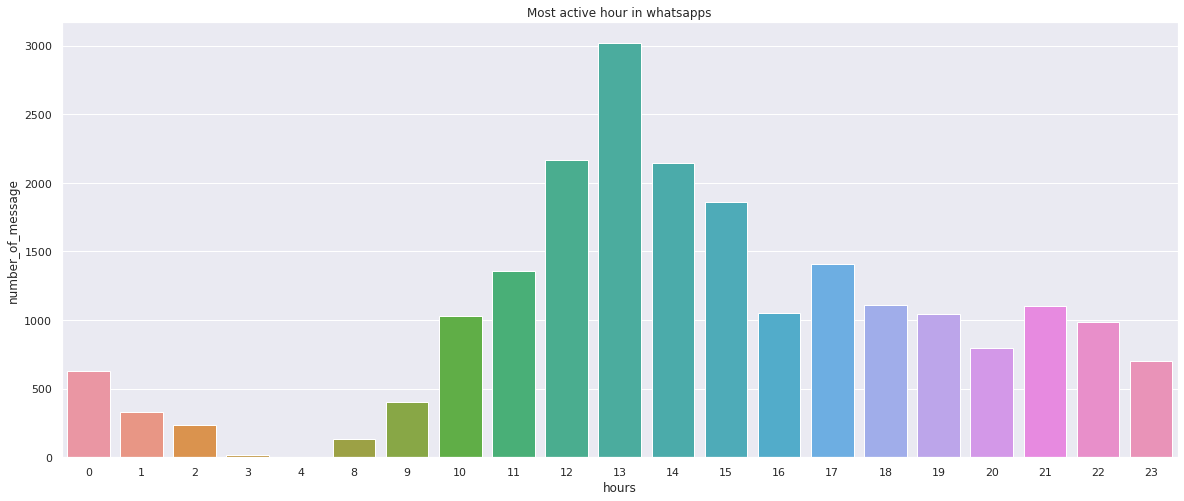## 4. Which month have the highest messages and also the busiest month?

This group was created between (05/02/2020–21/09/2020). Here we hope to found out the month that we are busiest and we look in the amount of message is generated.

In :
``````whatsapp_df4 = whatsapp_df.copy()
whatsapp_df4['Number_of_messages'] =  * whatsapp_df4.shape

whatsapp_df4['month'] = whatsapp_df4['datetime'].apply(lambda x: x.month)

df_month = whatsapp_df4.groupby('month')['Number_of_messages'].count().sort_values(ascending = False).reset_index()
``````
Out:

In this analysis, we found that the busiest month is on July (7) the total number of messages had reached around 7000, The reason behind it is because on that month we are all busy on University assignment and mid-term test. This show that the users are very active during that month. The following month you are able to see there is a decrease of chat. This is highly due to the user are too busy on mid-term and assignment due date.

Moreover, you are able to see there is no March(3). This is because during that period of time Malaysia goes through pandemic lockdown due to COVID-19. So the group had been silent until the University resume in E-learning mode. Because of e-learning now you are able to see there are an increase in April(4) and a drop on May (5) due to University semester break.

In :
``````#Formating
sns.set_style("darkgrid")

#The background of the chart
matplotlib.rcParams['font.size'] = 12
matplotlib.rcParams['figure.figsize'] = (12, 9)
matplotlib.rcParams['figure.facecolor'] = '#00000000'
fig, ax = plt.subplots()

#Creating a bar chart
sns.barplot(x=df_month.month,y=df_month.Number_of_messages ,hue='month',data=df_month,dodge=False,palette="pastel")
plt.title("Month that have the highest messages and the busiest month?")``````
Out:
``Text(0.5, 1.0, 'Month that have the highest messages and the busiest month?')``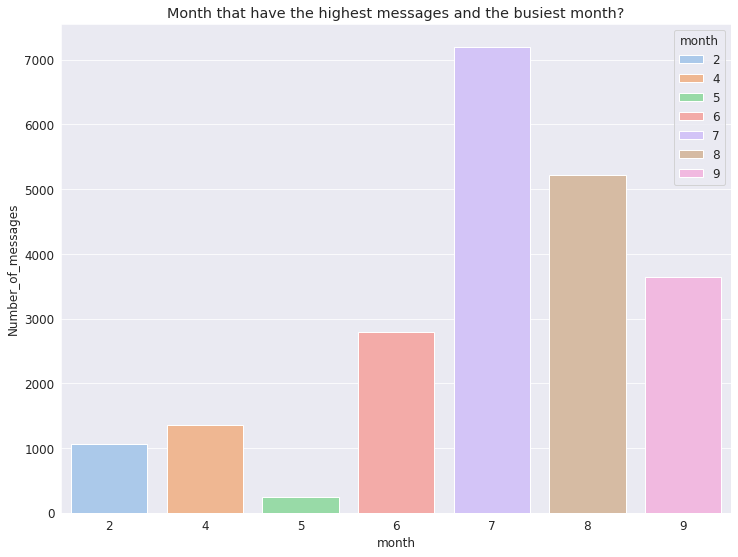## 5.Determine which word or text did the user use the most?

In here we are going to use a word cloud to visual representation of word in the chat and determine which word is widely use by the user? The reason behide this analysis is to understand the user behaviors. Why do we say so? Because if the word is repeating use we can say that the user will more likely to use the particular or text again in the other chat.

In :
``````whatsapp_df5 = whatsapp_df.copy()

``````
In :
``````#Each of the word in the message will be review
word = " ".join(review for review in whatsapp_df5.message)

stopwords = set(STOPWORDS)

#delete the word/text that are commonly used(eg.the,yes,no,bye,or and is)
stopwords.update(["the","is","yea","ok","okay","or","bye","no","will","yeah","I","almost","if","me","you","done","want","Ya"])

#Creating a word cloud
wordcloud = WordCloud(width = 500, height =500 ,stopwords=stopwords, background_color="black",min_font_size = 10).generate(word)

plt.figure( figsize=(10,5))
plt.imshow(wordcloud, interpolation='bilinear')
plt.axis("off")
plt.show()

``````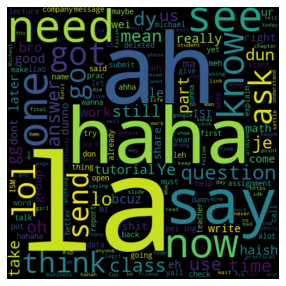In :
``wordcloud.to_image()``
Out: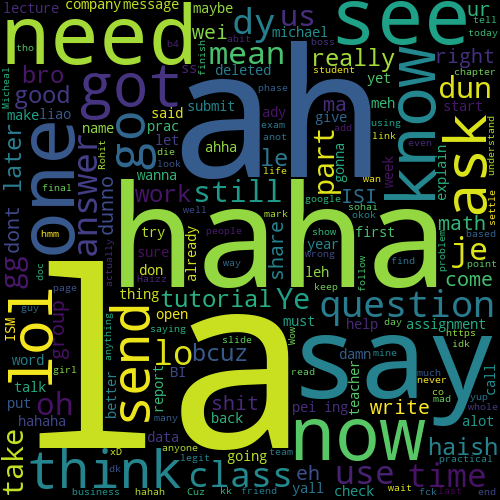In :
``jovian.commit(project=project_name,enviroment=None,files=["Chat.txt"])``
```[jovian] Attempting to save notebook.. [jovian] Updating notebook "edsenmichaelcy/whatsapp-chat-analysis-course-project" on https://jovian.ml/ [jovian] Uploading notebook.. [jovian] Capturing environment.. [jovian] Uploading additional files... [jovian] Committed successfully! https://jovian.ml/edsenmichaelcy/whatsapp-chat-analysis-course-project ```
Out:
``'https://jovian.ml/edsenmichaelcy/whatsapp-chat-analysis-course-project'``

## Conclusion

Data Retrieval

First we learn how to load data at whatsapp and understand the format as text file.Other than that we understand what kind format should we extract(eg "media" or "without media")

Data Preparation and Cleaning

In the data preparation and cleaning we learn on how to convert text file into dataframe by using txtTodf() functions. Then we learn to add name to the colums and even on how to clean image file from the dataframe

Then we start understand the business needed and the data in the dataframe. In the business understand how us to understand what question should we need to ask that will lead to business succeed.

Exploratory Data Analysis(EDA)

In the EDA we look at five important question to ask:

1. Which users have the most Chat/messages in the group?
2. Which emojis use the most by which users?
3. Most active hours?
4. Which month have the highest messages and also the busiest month?
5. Determine which word or text did the user use the most?

I really hope you are enjoying reading my notebook as well my medium post . Hope you like it and maybe you can try out your own data analysis too!!.

Thank you, Michael Chia Yin